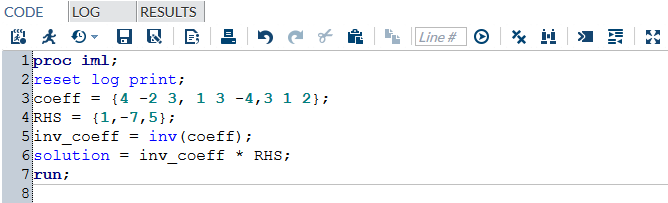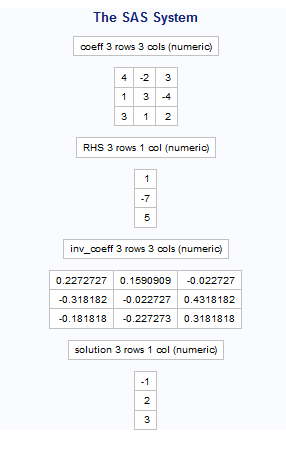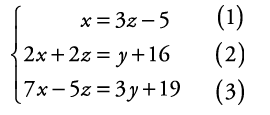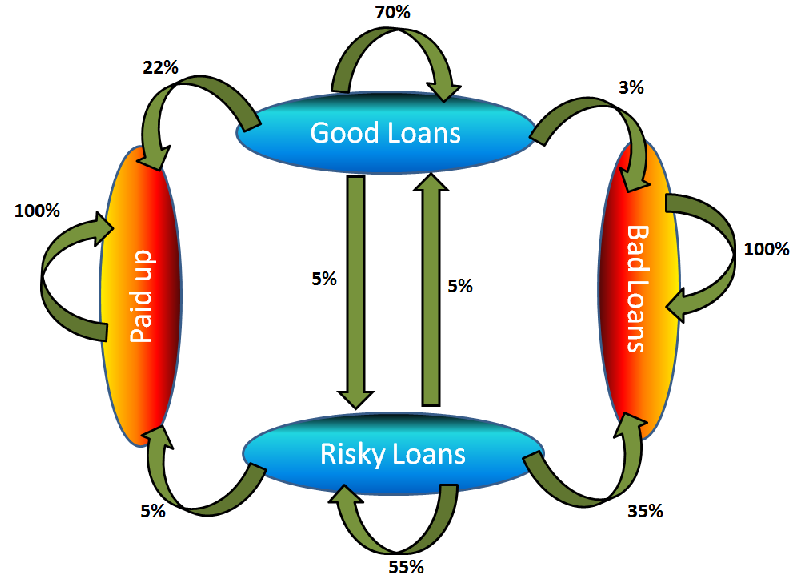Home » Applications of SAS Proc IML in Analytics

# Applications of SAS Proc IML in Analytics

In the last two articles on IML, we discussed basics of IML and how to perform various matrix operations using SAS IML. IML makes it really easy to work with matrices on SAS. This not only saves computational time but also makes matrix coding very efficient. This article will serve as a launch pad for implementing IML in your daily routine as an analyst. I have picked up some really simple examples to demonstrate how various commands on IML (discussed before) can be used in various scenarios:

### Application 1: Solve simultaneous equations:

Simultaneous equations can be solved efficiently using matrices. Following is a simple formulation of simultaneous equations :

Coefficient Matrix (A) * Independent Variable Matrix (X) = RHS Matrix (Y )

A * X =  Y

=>     Inv(A) * A * X = Inv(A) * Y

As Inv(A) * A = I (Shown in last article), we have

=>       X = Inv(A) * Y

#### Worked Out Example

Let us now consider an example to see the power of working with matrices. Following is a set of equations we will try solving, using SAS IML.Solving these equation becomes extremely simple by Matrices. Following is 4-line code which can do this job on IML.

proc iml;
reset log print;
coeff = {4 -2 3, 1 3 -4,3 1 2};
RHS = {1,-7,5};
inv_coeff = inv(coeff);
solution = inv_coeff * RHS;
run;Here is the final output :The results found using IML are X = -1 , Y = 2 , Z = 3.

#### Give this a try

Here is another example of 3 simultaneous equations you can give a try using IML.Once you have solved it, write the answer in the box below.

### Application 2: Solving Markov chains

If you would remember, I had discussed how Markov chains can be represented and solved using Matrix. If you need a refresher, have a look at these article:

Let us revisit the business case we used in the second article – Krazy Bank. Here is the transition diagram we had created:Can you write the IML code to compute the percentage of Bad Loans at end of year 1? Year 2? and finally compute the steady state scenario?

This should be a cake walk for those of you who have followed the article. Post your code in the comments below. I will post mine in a week’s time .

### End Notes:

As mentioned before, use of PROC IML can take use of SAS to a completely different level. I have demostrated 2 common applications for PROC IML. You can also apply IML procedures for Singular Value Decomposition (SVD) for dimensionality reduction or to transform your planes, while applying Support Vector Machines (SVM).

You now have all the tools to apply these algorithms in SAS using Matrix computations. This also concludes this series on PROC IML. Hopefully, you have a new tool in your armory.

### If you like what you just read & want to continue your analytics learning, subscribe to our emails, follow us on twitter or like our facebook page.

You can also read this article on our Mobile APP•wenyi says: